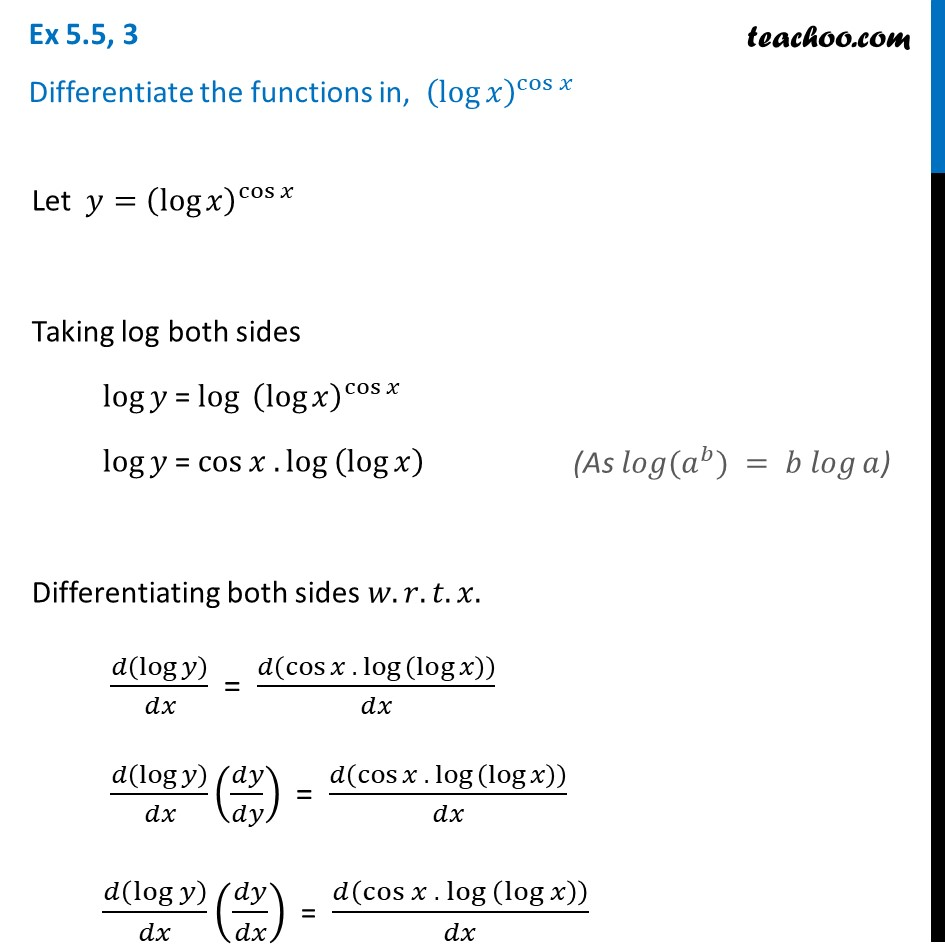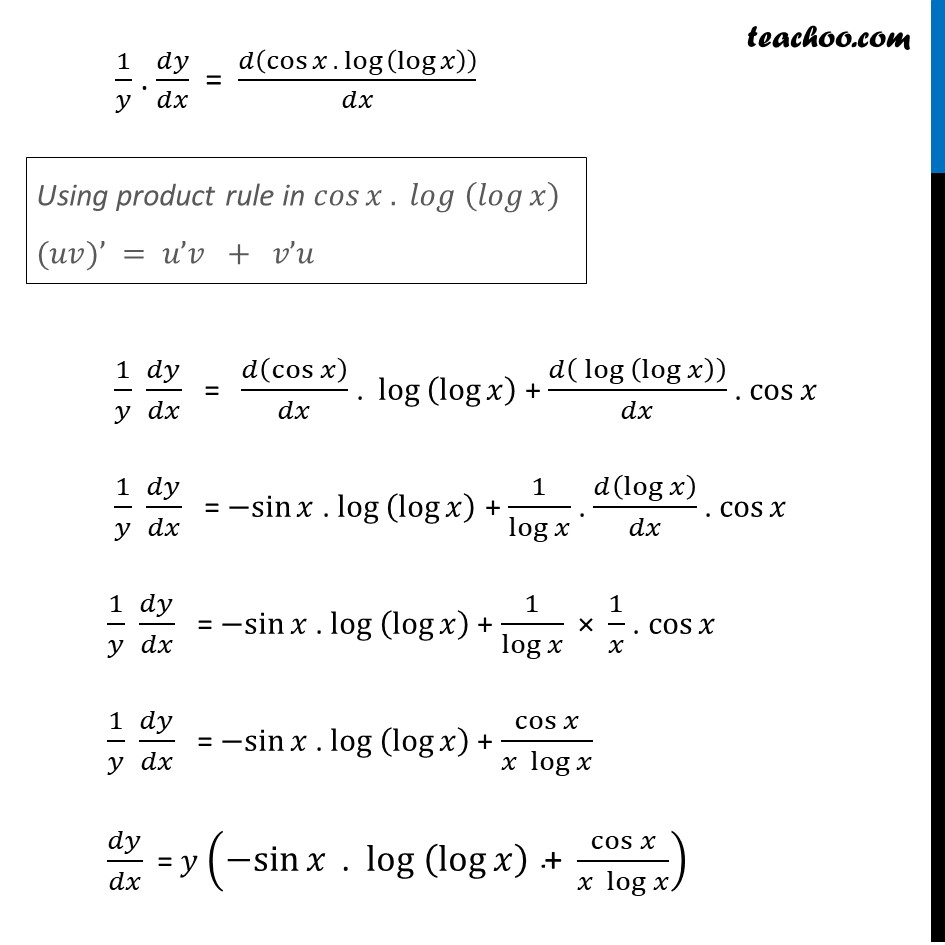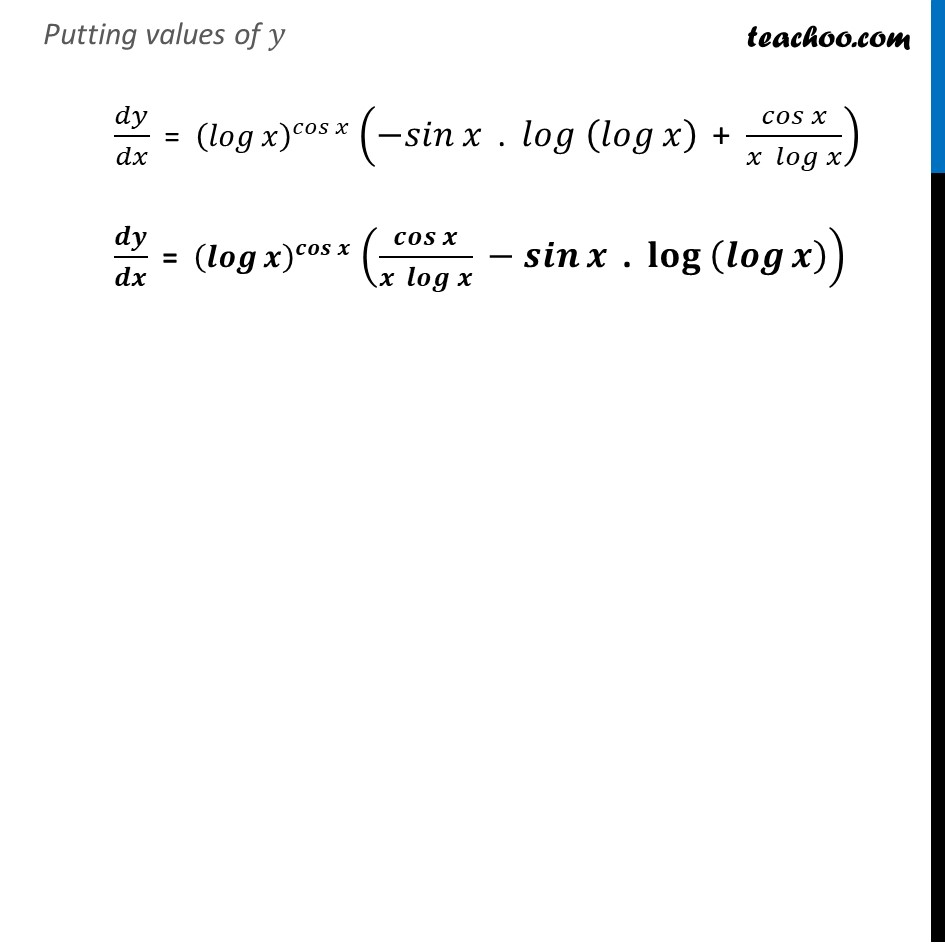Logarithmic Differentiation - Type 1

Chapter 5 Class 12 Continuity and Differentiability
Concept wiseLearn in your speed, with individual attention - Teachoo Maths 1-on-1 Class

### Transcript

Ex 5.5, 3 Differentiate the functions in, (log⁡𝑥 )^cos⁡𝑥 Let 𝑦=(log⁡𝑥 )^cos⁡𝑥 Taking log both sides log⁡𝑦 = log⁡〖〖 (log⁡𝑥 )〗^cos⁡𝑥 〗 log⁡𝑦 = cos⁡〖𝑥 .〖log 〗⁡(log⁡𝑥 ) 〗 Differentiating both sides 𝑤.𝑟.𝑡.𝑥. 𝑑(log⁡𝑦)/𝑑𝑥 = 𝑑(cos⁡〖𝑥 .〖 log 〗⁡(log⁡𝑥 ) 〗 )/𝑑𝑥 𝑑(log⁡𝑦 )/𝑑𝑥 (𝑑𝑦/𝑑𝑦) = 𝑑(cos⁡〖𝑥 .〖 log 〗⁡(log⁡𝑥 ) 〗 )/𝑑𝑥 𝑑(log⁡𝑦 )/𝑑𝑥 (𝑑𝑦/𝑑𝑥) = 𝑑(cos⁡〖𝑥 .〖 log 〗⁡(log⁡𝑥 ) 〗 )/𝑑𝑥 (As 𝑙𝑜𝑔⁡(𝑎^𝑏) = 𝑏 𝑙𝑜𝑔⁡𝑎) 1/𝑦 . 𝑑𝑦/𝑑𝑥 = 𝑑(cos⁡〖𝑥 .〖 log 〗⁡(log⁡𝑥 ) 〗 )/𝑑𝑥 1/𝑦 𝑑𝑦/𝑑𝑥 = 𝑑(cos⁡𝑥 )/𝑑𝑥 . 〖 log 〗⁡(log⁡𝑥 ) + 𝑑(〖 log 〗⁡(log⁡𝑥 ) )/𝑑𝑥 . cos⁡𝑥 1/𝑦 𝑑𝑦/𝑑𝑥 = 〖−sin〗⁡𝑥 . 〖log 〗⁡(log⁡𝑥 ) + 1/log⁡𝑥 . 𝑑(log⁡𝑥 )/𝑑𝑥 . cos⁡𝑥 1/𝑦 𝑑𝑦/𝑑𝑥 = 〖−sin〗⁡𝑥 . 〖log 〗⁡(log⁡𝑥 ) + 1/log⁡𝑥 × 1/𝑥 . cos⁡𝑥 1/𝑦 𝑑𝑦/𝑑𝑥 = 〖−sin〗⁡𝑥 . 〖log 〗⁡(log⁡𝑥 ) + cos⁡𝑥/(𝑥 log⁡𝑥 ) 𝑑𝑦/𝑑𝑥 = 𝑦 (〖−sin〗⁡𝑥 " . " 〖log 〗⁡(log⁡𝑥 )" + " cos⁡𝑥/(𝑥 log⁡𝑥 )) Using product rule in 𝑐𝑜𝑠⁡〖𝑥 .〖 𝑙𝑜𝑔 〗⁡(𝑙𝑜𝑔⁡𝑥 ) 〗 (𝑢𝑣)’ = 𝑢’𝑣 + 𝑣’𝑢 Putting values of 𝑦 𝑑𝑦/𝑑𝑥 = (𝑙𝑜𝑔⁡𝑥 )^𝑐𝑜𝑠⁡𝑥 (〖−𝑠𝑖𝑛〗⁡𝑥 " . " 〖𝑙𝑜𝑔 〗⁡(𝑙𝑜𝑔⁡𝑥 )" + " 𝑐𝑜𝑠⁡𝑥/(𝑥 𝑙𝑜𝑔⁡𝑥 )) 𝒅𝒚/𝒅𝒙 = (𝒍𝒐𝒈⁡𝒙 )^𝒄𝒐𝒔⁡𝒙 (𝒄𝒐𝒔⁡𝒙/(𝒙 𝒍𝒐𝒈⁡𝒙 ) 〖 − 𝒔𝒊𝒏〗⁡𝒙 " . " 〖𝐥𝐨𝐠 〗⁡(𝒍𝒐𝒈⁡𝒙 ) )